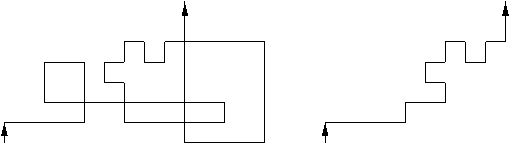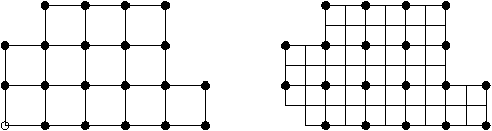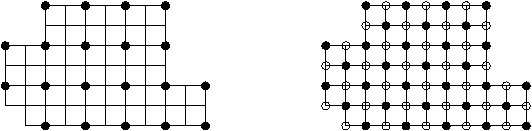Loop-Erased Random Walks

Richard Kenyon

Université de Paris-Sud

Algorithms Seminar

May 31, 1999

[summary by Cyril Banderier]

A properly typeset version of this document is available in postscript and in pdf.

If some fonts do not look right on your screen, this might be fixed by configuring your browser (see the documentation here).

Abstract
The loop-erased random walk is the simple curve obtained by removing in the chronological order the loops of the original random walk. A basic aspect of these walks in Z2 is studied: its average length (thus solving a conjecture of Guttmann). The techniques are combinatorial and use a bijection due to Temperley between maximal trees and perfect coupling.Figure 1: A random walk and its associated LERW

## 1   LERW=ST=DT

This is not a new equality between new complexity classes but it simply emphasizes the fact that loop-erased random walk (LERW), spanning tree (ST) and domino tiling (DT) are essentially the same object.

Indeed, in  shows that, for a uniformly chosen spanning tree on a region of Z2, the unique arc (branch) between two points has the same distribution as the LERW between these two points. Moreover, Temperley  gives a constructive one-to-one correspondence between spanning trees and domino tilings.

From the other talk of Richard Kenyon, we know that domino tiling (the so-called two dimensional lattice dimer model, a model which has some ties with Ising model) is the only nontypical ad hoc statistical physical model where conformal invariance is proved, so representations of the Virasoro algebra  could help finding critical exponents.

In order to solve this ``self-avoiding walk model'' (i.e., to set the critical exponent), R. Kenyon does not use representation theory, but a ``discrete Laplacian'', from which he gets the full asymptotics. Whereas a lot is known about properties of the continuous Laplacian , works on the discrete Laplacian are more recent .

As conjectured by Bursill and Guttmann , the exponent for LERW is 5/4. Richard Kenyon proves this by applying the ``equality'' LEWR=ST on the following theorem
Theorem 1   On the uniform spanning tree process on N×Z, the expected number of vertices on the arc from (0,0) to ¥ which lie within distance N of the origin is N5/4+o(1).
The next theorem is sometimes attributed to Kirschoff  and proven in .
Theorem 2  [Matrix-tree Theorem]   For a graph G with set of vertices {vi}, let
D:=
æ
ç
ç
ç
ç
è
 deg(v1) 0 0 0 ·   ·   · 0 0 0 deg(vn)
ö
÷
÷
÷
÷
ø
then the number of spanning trees of G is the product of the nonzero eigenvalues of D divided by the size of G. For an m× n rectangle, the number of spanning trees is thus

Õ
 (j,k)¹ 0 j=0,..., m-1 k=0,..., n-1
æ
ç
ç
è
4-2cos(
p k
n
)-2cos(
p j
m
) ö
÷
÷
ø
.

It is possible to extend this kind of result to a class of polygons which are decomposable in rectangles, the so-called Temperleyan polyominoes. (Triangulations are also a way, as there is a determinant-like expression for triangles.)Figure 2: A graph and its associated Temperleyan polyomino

The number of spanning trees of the graph is the number of domino tilings of its Temperleyan polyomino. Taking the log in the previous formula gives a special case of the following theorem:

Theorem 3   Let UÌ R2 be a rectilinear polygon with V vertices. For each e>0, let Pe be a Temperleyan polyomino in eZ2 approximating U in the natural sense (the corners of pe are converging to the corners of U). Let Ae be the area and Perime be the perimeter of Pe. Then the log of the number of domino tilings of Pe is
c0 A
 e
e2
+
c1 Perim
 e
e
+O(log(e))
where c0=G/p, G=1-1/32+1/52-··· is Catalan's constant, c1=G/2p+log((2)1/2 -1)/4.

According to the author, ``Part of the motivation for the above theorem is to validate a certain heuristic, which attempts to explain how the presence of the boundary affects the long-range structure of a random tiling. In particular it attempts to explain how the boundary affects the densities of local configurations far from the boundary . This heuristic is called the `phason strain' principle. The heuristic is as follows: The boundary causes the average height function of a tiling to deviate slightly from its entropy-maximizing value of 0. At a point in the region where the average height function has nonzero slope, the ``local'' entropy there is smaller than the maximal possible entropy, by an amount proportional to the square of the gradient of the average height function. The system behaves in such a way as to maximise the total entropy subject to the given boundary values of the height function, and the resulting average height function is the function which minimises the (integral of) the square gradient. That is, the average height function is harmonic.''

Anyway an exact application of the phason strain principle gives in fact slightly different asymptotics (from the one given in the theorem 3), so this principle is not totally valid here, but however it gives a good approximation.

## 2   Height Function

For a given tiling, the height function h is easily defined  by bicolouring the Temperleyan polyomino (there are no adjacent vertices of the same colour):Figure 3:                 A Temperleyan polyomino and the bicoloured associated graph

then, for each oriented edge AB on the border of a domino,
h(A)-h(B):=
ì
í
î
 +1 if the square on the left of AB is black, -1 otherwise.

Note that, up to an arbitrary additive constant, the height of the boundary is independent of the tiling.

For a smaller and smaller lattice (e.g., e Z2), one can approximate any domain U of C. For a very fine lattice (in fact, taking the limit when e® 0), one can study the probability of appearance in the tiling of some patterns, their repartition in the tiling and also how the shape of the boundary influences the tiling. It appears that there are links with conformal theory, as explained below.

Let Pe be a Temperleyan polyomino associated to a rectilinear polygon U of C. Now, let he be the average height function of Pe, that is the average height over all domino tilings of Pe and then define
h(x):=
 lim e ® 0
h
 e
(x
 e
).
For xÎ U, h(x) is defined by continuity from values of h in the interior.

The remarkable fact is that this limiting average height function h can be expressed as
h(v)=
4
p
Á ó
õ
 v b0
 lim z® v
æ
ç
ç
è
F+(v,z)-
2
p(z-v)
ö
÷
÷
ø
du=-
2
p
Á log Ã'(z),
where Ã is the Weiertrass elliptic function and where F+(u,zdu is a meromorphic 1-form, thus allowing some links with conformal mapping theory. We refer to ``Conformal invariance of domino tiling'' (1997) and to ``The asymptotic determinant of the discrete Laplacian'' (1999) for further informations1.

## References


Brooks (R. L.), Smith (C. A. B.), Stone (A. H.), and Tutte (W. T.). -- The dissection of rectangles into squares. Duke Mathematical Journal, vol. 7, 1940, pp. 312--340.


Burton (Robert) and Pemantle (Robin). -- Local characteristics, entropy and limit theorems for spanning trees and domino tilings via transfer-impedances. The Annals of Probability, vol. 21, n°3, 1993, pp. 1329--1371.


Destainville (N.), Mosseri (R.), and Bailly (F.). -- Configurational entropy of codimension-one tilings and directed membranes. Journal of Statistical Physics, vol. 87, n°3-4, 1997, pp. 697--754.


Guttmann (A.) and Bursill (R.). -- Critical exponent for the loop-erased self-avoiding walk by Monte-Carlo methods. Journal of Statistical Physics, vol. 59, 1990, pp. 1--9.


Kac (Mark). -- Can one hear the shape of a drum? American Mathematical Monthly, vol. 73, n°4, part II, 1966, pp. 1--23.


Kenyon (Richard). -- Rigidity of planar tilings. Inventiones Mathematicae, vol. 107, n°3, 1992, pp. 637--651.


Kenyon (Richard). -- Tiling a polygon with parallelograms. Algorithmica, vol. 9, n°4, 1993, pp. 382--397.


Kenyon (Richard). -- Local statistics of lattice dimers. Annales de l'Institut Henri Poincaré. Probabilités et Statistiques, vol. 33, n°5, 1997, pp. 591--618.


Kenyon (Richard). -- Tilings of convex polygons. Annales de l'Institut Fourier, vol. 47, n°3, 1997, pp. 929--944.


Kenyon (Richard). -- Tilings and discrete Dirichlet problems. Israel Journal of Mathematics, vol. 105, 1998, pp. 61--84.


Kirchhoff (G.). -- Über die Auflösung der Gleichungen, auf welche man bei der Untersuchung der linearen Verteilung galvanischer Ströme geführt wird. Annalen für der Physik und der Chemie, vol. 72, 1847, pp. 497--508.


Pemantle (Robin). -- Choosing a spanning tree for the integer lattice uniformly. The Annals of Probability, vol. 19, n°4, 1991, pp. 1559--1574.


Temperley (H. N. V.). -- Enumeration of graphs on a large periodic lattice. In Combinatorics. London Mathematical Society Lecture Note Series, pp. 155--159. -- Cambridge University Press, 1974. Proceedings of the British Combinatorial Conference, University College, Wales, Aberystwyth, 1973.


Thurston (William P.). -- Conway's tiling groups. The American Mathematical Monthly, vol. 97, n°8, 1990, pp. 757--773.


Virasoro (M. A.). -- Physical Review D. (3), 1970, pp. 2933--2936.

1
Like other recent preprints of the author, they are available at his home page
http://topo.math.u-psud.fr/~kenyon/

This document was translated from LATEX by HEVEA.